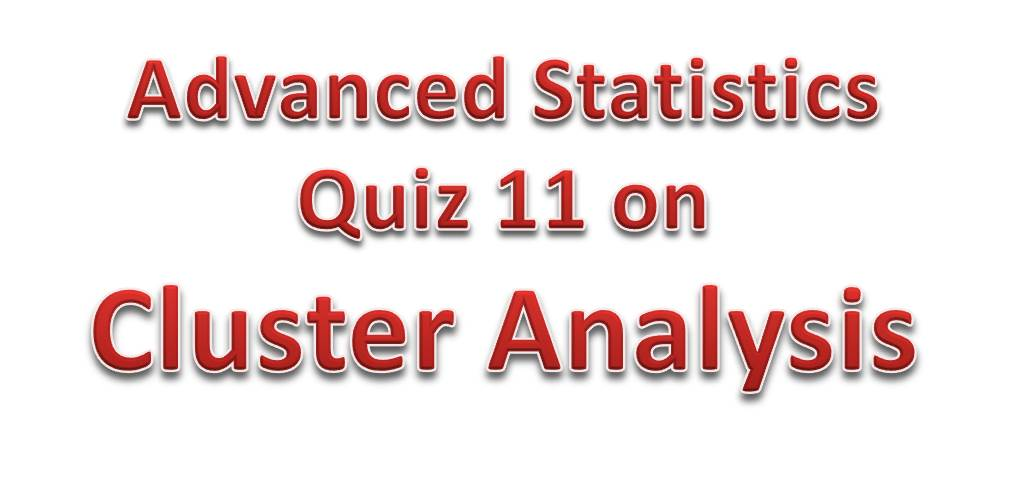# Advanced Statistics Quiz 11 – Cluster AnalysisGood to see you here!
Today’s quiz would be based on Cluster Analysis. So let’s get started.

Question 1: What is Cluster Analysis?
Cluster Analysis is the statistical procedure that is aimed at grouping data object based on the information found in the data set that describes the objects and their attributes

Question 2: What is the Goal of Cluster Analysis?
The objective of cluster analysis is to group objects with similar characteristics into one cluster.

Question 3: What are the two types of Clustering?
The two types of clustering are:
Hierarchical Clustering: Clusters are arranged in a hierarchical tree
Partitioning Clustering: Data are grouped into distinct subsets that does not overlap

Question 4: Describe the k-Means Clustering
K-Means clustering is a partitioning clustering approach where each cluster is  associated with a centroid or center point and each data point is assigned to  the centroid that is closest to it. The number of clusters is specified in advance.

Question 5: Write the k-Means Clustering Algorithm?
i. Choose the initial value of K
ii. repeat
iii. Form K clusters by assigning each point to the closest centroid
iv. Recalculate the centroid of each cluster
v. Move the centroid to the new computed position
vi. until The centroids position don’t change

Question 6: How do you Choose Initial Value of K for k-Means Clustering

• Use another clustering method to estimate it
• Run the algorithm with different values of K and then choose the one that is optimal
• Use the prior knowledge about the characteristics of the data

Question 7: How do you choose the centroid for the cluster?

• Random selection from the feature space
• Random selection from the data set
• Look for dense regions of space
• Space them uniformly around the feature space

Question 8:  How is the quality of a cluster measured?

• The size of the cluster vs the distance between the clusters
• The Distance between members of the clusters
• The Diameter of the smallest sphere

Question 9: What are some limitations of k-Means Clustering?
Not efficient if data contains outliers
Fails for non-convex round clusters

Question 9: What is McQueen’s Algorithm used for?
The McQueen’s Algorithm is used for measuring the goodness of the clustering and for minimizing the compactness function in finite steps

Question 10: Outline and explain the two types of Hierarchical Clustering
The two types of hierarchical clustering are:
Top-Down Clustering
Bottom-Top Clustering

How Bottom-Top or Agglomerative Clustering work

• Start with each of the data points in its own cluster
• Merge two clusters that are similar
• Repeat the merging until there is a single cluster of all he data points

How Top-Down or Divisive Clustering Work

• Remove the data point that seems to far away from other points
• Repeat the process until all points is in its own cluster

Question 11: Mention three ways to compute dissimilarity between clusters

• Group Average

Question 12: Compare k-Means and Hierarchical Clustering
k-Means produces single partition while hierarchical produces different partitions
k-Means needs the number of clusters specified in advance while hierarchical does not
k-Means is have a more efficient run-time than the hierarchical

Question 13: What is a Dendrogram?
A dendrogram is a tree diagram used to illustrate the arrangement of clusters in hierarchical clustering.

I would stop here so I can allow you some time to get your head around these concepts.